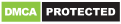# Learning Math :Add , Subtract , Multiply & Divide

Genres
Version
4.7
Developer
-
Requires
Android 4.4+
Updated
2020-05-23★ Free Math learn Games for everyone Best maths practice game in 2020 to train your brain & is designed for all ages

★ maths quiz is one of the greatest math gams in education math gams category
Smallest in size Maths App learn quiz on Google Play ! Easiest addition and multiplication and division games with Addition and Subtraction games all in one app.

★FREE practice mode to Learn Mathematics all essentials of arithmetic operations math gams and solve unlimited number of Maths problems comprising of two-digit numbers with/without carry-overs for addition, borrows for subtraction and divise Plus, FREE starter worksheets to test addition problems.
★you can have fun with this math gams “fun maths gams” with infinity math operations all this for free

★With this app you’ll just pick a number from 1 to 12 int beginner (easy) level and 1 to 50 in medium level and 1 to 100 in hard level and you’ll get its corresponding table so that you can memorize Addition , Subtraction , Multiplication , Division,square,square root,square cube.all this in one math gams “learn maths”
Languages support: English, French,arabic,italian
★ This game is to learn square and cube and square root table from 1 to 100. Its nice for students in school.
– maths learner support Table Pronunciation options

Features :
– math times tables with infinity math numbers
– math multiplication , square , square cube , square cube root in one math gams for free
– math app quiz and test with intelligent ways to learn and smart design to get The best experience
– math flashcards with different math level : easy ,medium and hard level “Professional level ” in all math operations : addition , multiplication , division and subtraction
-math easy and speed to learn supporting tree languages Arabic , French and English and we work now to support all languages
– square table, square cube root table learn and quiz as exam to share your score with your friends and play with them
– Division games: Practice and learn Division tables, Subtraction games: Subtracting numbers to solve the equations , Multiplication games: Multiplication tables learning and duel play mode and Addition games: Adding numbers with Quiz and Practice games
– educational mathematical quiz game and math learn
-Add other mathematical operations such as square table, square cube, cube root ,and square roots
-A brand new design gives you a very pleasant experience
– Share the results with your friends
– the new pronunciation property of mathematical operations in English and French to improve your math skills
-Full control of game settings

Recommended for you

You may also like